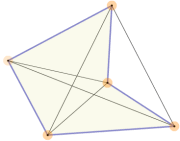# Diagonals of PolygonsA square has 2 diagonals An octagon has 20 diagonals

A polygon's diagonals are line segments from one corner to another (but not the edges).

The number of diagonals of an n-sided polygon is:

n(n − 3) / 2

### Examples:

• a square (or any quadrilateral) has 4(4−3)/2 = 4×1/2 = 2 diagonals
• an octagon has 8(8−3)/2 = 8×5/2 = 20 diagonals.
• a triangle has 3(3−3)/2 = 3×0/2 = 0 diagonals.

### Try it Yourself:A diagonal can actually be outside the polygon, which happens with some concave polygons.